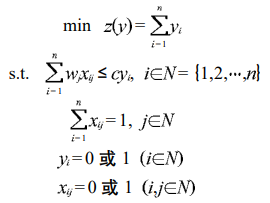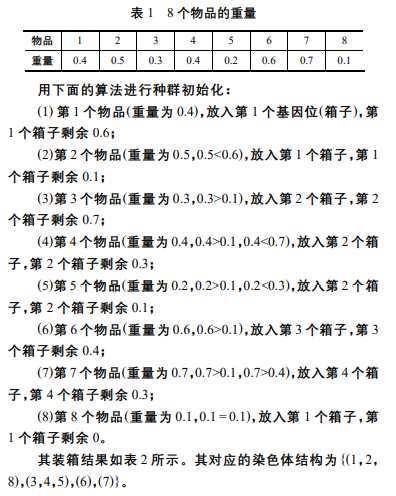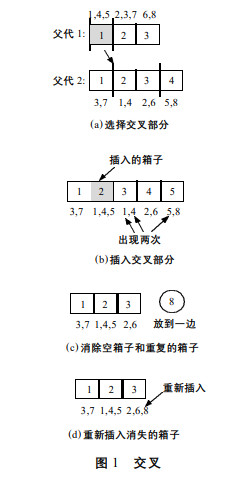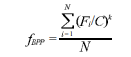• 装箱或背包问题？ (或者遗传算法解决)
2021-05-25 00:40:50

共有三个箱子分别容量为200、500、1000 ;物品有四个重量分别为150、200、300、800;

要求一次装箱把所有物品装完、箱子可以有空的，请问一共有多少种算法？

输出例子：

第一种装箱 ：  箱子：1000         500             200        利用率：90%

物品：800+200   300+150     0

第二种装箱 ：  箱子：1000         500             200        利用率：95%

物品：800+150   300+200     0

第三种装箱 ：  箱子：1000         500             200        利用率：78%

物品：800           300+200     150

第四种装箱 ：  箱子：1000         500             200        利用率：85%

物品：800           300+150     200

更多相关内容
• 装箱问题遗传算法MATLAB求解。希望对你有用。
• 该项目包含使用遗传算法解决装箱问题的解决方案。 该项目中的代码是作为2007年南里奥格兰德州联邦大学（UFRGS-巴西）组合优化课程中问题的解决方案而创建的。开源软件
• 装箱问题遗传算法MATLAB实现.doc，这份文档介绍了装箱问题遗传算法MATLAB实现，装箱问题遗传算法MATLAB实现.docmatlab
• 集装箱配载是一个复杂的组合...在满足一定的约束条件下,提出一种3维集装箱装载的自适应遗传算法.算法中考虑了货物放置方向和装载容积等约束条件,给出了有效的解码算法.实例仿真结果表明了该算法的有效性和实用性.</p>
•matlab
• 问题描述： n个重量不同的物体放入k个有重量限制的箱子。 ** 数学公式表达： 编码方式： ①基于箱子的编码表示。用基因的位置表示物品，基因的值表示该物品所放入的箱子； ②基于物品的编码表示。只对物品的排列...

问题描述：
n个重量不同的物体放入k个有重量限制的箱子。
**
数学公式表达：编码方式：
①基于箱子的编码表示。用基因的位置表示物品，基因的值表示该物品所放入的箱子；
②基于物品的编码表示。只对物品的排列进行编码，然后应用解码器得到其对应的解。

染色体结构：基因的位置代表箱子，基因的值代表所要放入的所有物品。

种群初始化类似贪心，装到装不下为止。双亲双子交叉算子，
步骤：
步骤 1 从两个父代中各随机选择两个交叉位置，从而对 每个父代选定交叉部分。

步骤 2 将第 1 个父代交叉部分的内容插入到第 2 个父 代第 1 个交叉位置之前。由于交叉对染色体的部分群体进行 操作，这就意味着从第 1 个父代插入一些群体(箱子)到第 2 个 父代中。

步骤 3 从产生的后代中，去掉所有装有重复出现的物品 的箱子，并使得这些物品原先的从属关系让位于“新”插入的 箱子。如果有消失的箱子，则用优先配合启发式方法 (first-fit heuristic，FFH)将物品放入箱子中。
FFH 是指将每个物品放入 其能够放入的最小下标的已初始化箱子中，如果当前物品无 法放入任何已初始化的箱子，则放入一个新箱子。

步骤 4 改变两个父代的角色并重新应用第 2 步到第 3 步生成第 2 个子代。本来我也没看懂这个交叉，但是这是双亲，应该就能理解了。

变异算子：
变异的基本策略为随机消除一个已经使用的箱子。消除的箱子中的物品用上述 FFH 启发式方法插入染色体中。

适应值函数式中： N——染色体中使用的箱子数量， Fi——第 个箱子中所有物品的重量之和(箱子的填充程度)，C是箱子的重量限制， k是常数( k>1)。常数k表示了对装得满的箱子的重视程度。 箱子填的越满,k越大。根据计算经验，k = 2 得到的结果较好。 N越小 (使用的箱子数量越少)，Fi越大 (箱子填的越满)， fbpp就越大。

展开全文人工智能
• 集装箱配载是一个复杂的组合...在满足一定的约束条件下,提出一种3维集装箱装载的自适应遗传算法。算法中考虑了货物放置方向和装载容积等约束条件,给出了有效的解码算法。实例仿真结果表明了该算法的有效性和实用性。
• 考虑体积重量的装箱问题（贪婪策略装箱）—— 基于遗传算法 1 装箱问题简介 经典装箱问题要求把一定数量的物品放入容量相同的箱子中，在满足每个箱子装载物品的大小之和不超过箱子容量的约束下，最小化箱子数目。...

# 考虑体积重量的装箱问题（贪婪策略装箱）—— 基于遗传算法

## 1 装箱问题简介

经典装箱问题要求把一定数量的物品放入容量相同的箱子中，在满足每个箱子装载物品的大小之和不超过箱子容量的约束下，最小化箱子数目。装箱问题是复杂的离散组合最优化问题，属于NP完全问题。

## 2 场景设计

已知货物的重量和体积，在满足箱子载重和容积约束的情况下，最小化箱子使用个数，并除了最后一个箱子外，前面的箱子尽量装满。

## 3 遗传算法设计

### 3.1 算子设计

采用自然数编码、锦标赛选择、顺序交叉、基本位变异、一对一生存者竞争。
染色体的自然数编码如下图所示，采用贪婪策略进行编码。### 3.2 适应度设计

Fit = 总体积/(已使用车辆容积和+最后一辆车使用的容积)*总重量/(已使用车辆载重和+最后一辆车使用的载重)

这里适应度设计的目的在于让前面先装的箱子尽量装满，也就是在最小化箱子数的大目标下，使最后一辆车尽量少装货物。由于有两个参数–体积和质量，这里简单运用乘积进行平衡，可能不是最优的，但会是比较接近最优的。

### 3.3 遗传算法实现

# -*- coding: utf-8 -*-
import pandas as pd
import random

def tournament_select(pops,popsize,fit,tournament_size):
new_pops = []
while len(new_pops)<len(pops):
tournament_list = random.sample(range(0,popsize),tournament_size)
tournament_fit = [fit[i] for i in tournament_list]
#转化为df方便索引
tournament_df = pd.DataFrame([tournament_list,tournament_fit]).transpose().sort_values(by=1).reset_index(drop=True)
new_pop = pops[int(tournament_df.iloc[0,0])]
new_pops.append(new_pop)

return new_pops

def crossover(popsize,parent1_pops,parent2_pops,pc):
child_pops = []
for i in range(popsize):
parent1 = parent1_pops[i]
parent2 = parent2_pops[i]
child = [-1 for i in range(len(parent1))]

if random.random() >= pc:
child = parent1.copy()#随机生成一个
random.shuffle(child)

else:
#parent1
start_pos = random.randint(0,len(parent1)-1)
end_pos = random.randint(0,len(parent1)-1)
if start_pos>end_pos:start_pos,end_pos = end_pos,start_pos
child[start_pos:end_pos+1] = parent1[start_pos:end_pos+1].copy()
# parent2 -> child
list_index = list(range(end_pos+1,len(parent2)))+list(range(0,start_pos))
j = -1
for i in list_index:
for j in range(j+1,len(parent2)):
if parent2[j] not in child:
child[i] = parent2[j]
break

child_pops.append(child)
return child_pops

def mutate(pops,pm):
pops_after_mutate = []
mutate_time = 0
for i in range(len(pops)):
pop = pops[i].copy()
if random.random() < pm:
while mutate_time<3:
mut_pos1 = random.randint(0,len(pop)-1)
mut_pos2 = random.randint(0,len(pop)-1)
if mut_pos1 != mut_pos2:pop[mut_pos1],pop[mut_pos2] = pop[mut_pos2],pop[mut_pos1]
mutate_time += 1
pops_after_mutate.append(pop)

return pops_after_mutate

def package_calFitness(cargo_df,pop,max_v,max_m):
'''
输入：cargo_df-货物信息,pop-个体,max_v-箱子容积,max_m-箱子在载重
输出：适应度-fit，boxes-解码后的个体
'''
box_num = 0#装满的箱子数
v_sum,m_sum = 0,0
v,m = 0,0
boxes,box = [],[]
for j in pop:
v_j = cargo_df[cargo_df['货物序号']==j]['体积'].iloc
m_j = cargo_df[cargo_df['货物序号']==j]['重量'].iloc
v += v_j
m += m_j
if (v_sum+v_j <= max_v) and (m_sum+m_j <= max_m):
box.append(j)
v_sum = v_sum+v_j
m_sum =m_sum+m_j
else:
boxes.append(sorted(box))
box_num += 1
box = []
box.append(j)
v_sum = v_j
m_sum = m_j

#最后一个箱子
boxes.append(sorted(box))

#fit=总体积/(已使用车辆容积和+最后一辆车使用容积)*总重量/(已使用车辆载重和+最后一辆车使用载重)
fit = (v/(v_sum+box_num*max_v))*(m/(m_sum+box_num*max_m))
return round(fit,4),boxes

def package_GA(cargo_df,generations,popsize,tournament_size,pc,pm,max_v,max_m):
#初始化种群
cargo_list = list(cargo_df['货物序号'])
pops = [random.sample(cargo_list,len(cargo_list)) for i in range(popsize)]#种群初始化
fit,boxes = [-1]*popsize,[-1]*popsize

for i in range(popsize):
fit[i],boxes[i] = package_calFitness(cargo_df,pops[i],max_v,max_m)

best_fit = max(fit)
best_pop = pops[fit.index(max(fit))]
best_box = boxes[fit.index(max(fit))]

if best_fit==1:return best_pop#1说明除最后一辆车都装满，已是最优解

iter = 0#迭代计数
while iter < generations:
pops1 = tournament_select(pops,popsize,fit,tournament_size)
pops2 = tournament_select(pops,popsize,fit,tournament_size)
new_pops = crossover(popsize,pops1,pops2,pc)
new_pops = mutate(new_pops,pm)
iter += 1
new_fit,new_boxes = [-1]*popsize,[-1]*popsize#初始化
for i in range(popsize):
new_fit[i],new_boxes[i] = package_calFitness(cargo_df,new_pops[i],max_v,max_m)#计算适应度
for i in range(len(pops)):
if fit[i] < new_fit[i]:
pops[i] = new_pops[i]
fit[i] = new_fit[i]
boxes[i] = new_boxes[i]

if best_fit < max(fit):#保留历史最优
best_fit = max(fit)
best_pop= pops[fit.index(max(fit))]
best_box = boxes[fit.index(max(fit))]

print("第",iter,"代适应度最优值：",best_fit)
return best_pop,best_fit,best_box

if __name__ == '__main__':
#数据
num = list(range(100))#货物编号
volumns = [1,6,7,8,1,2,3,1,8,8,10,1,9,3,4,3,5,7,4,6,5,5,9,5,6,3,9,9,6,3,4,2,1,3,5,9,6,6,8,5,6,2,7,9,5,1,7,5,10,6,
4,6,9,7,2,4,3,7,5,4,5,10,2,1,4,10,9,6,10,10,10,2,10,2,4,6,4,1,7,6,1,10,1,3,4,1,7,3,6,5,3,10,6,8,1,6,4,4,10,3]#体积
weight = [3,5,3,8,10,4,7,2,10,1,9,2,1,9,7,1,7,1,4,2,5,9,1,6,1,4,2,1,2,1,5,5,6,8,3,6,7,4,9,7,7,4,8,3,9,4,1,1,9,5,8,
4,10,3,5,1,7,8,8,2,8,7,1,10,3,3,8,2,4,6,8,3,5,8,10,5,7,5,7,1,9,1,5,9,9,2,10,2,9,3,7,10,5,1,2,1,9,8,6,9]#重量
cargo_df = pd.DataFrame({'货物序号':num, "体积":volumns, "重量":weight})

M,V= 100,100 #箱子载重容积

#GA参数
generations = 50
popsize = 40
tournament_size = 4
pc = 0.9
pm = 0.1

pop,fit,box = package_GA(cargo_df,generations,popsize,tournament_size,pc,pm,V,M)
print("最优解：",box)



### 3.4 结果

最优解： [[0, 1, 6, 9, 14, 17, 21, 23, 24, 25, 31, 51, 52, 53, 57, 59, 82, 88, 98], [2, 5, 10, 12, 26, 34, 37, 38, 40, 54, 55, 64, 77, 78, 83, 87, 90, 94, 96, 97], [18, 19, 28, 43, 45, 47, 50, 56, 58, 60, 63, 67, 70, 71, 76, 79, 80, 81, 93, 99], [11, 13, 16, 29, 30, 32, 33, 35, 36, 39, 41, 46, 48, 49, 69, 75, 91, 95], [3, 4, 7, 8, 20, 22, 27, 42, 44, 62, 66, 68, 72, 73, 74, 85, 92], [15, 61, 65, 84, 86, 89]]
，总共需要6个箱子，箱子货物体积分别是[100, 98, 99, 97, 97, 39]，重量分别是[96, 100, 98, 99, 97, 33]。

## 4 总结

装箱问题的目标是最小化箱子使用数量，在实际应用中，可能会有更细化的一个目标，比如先装货物的箱子尽量装满（最后一个箱子尽量少装），或者平衡所有箱子的载重和容积，这里实现的差别主要在于编码和适应度设计。本文求解的问题是尽量将前面先装的箱子装满，使用遗传算法进行求解，求解速度还是有点慢，不过从适应度上看求解效果应该还是不错的。
记录学习过程，欢迎指正

展开全文python
• 遗传算法解决资源分配问题，翟旭，，资源分配问题是一种特殊的装箱问题，其实质是在生产资料数量既定的条件下，如何选择生产部门，确定生产规模，寻求产品与产品之间
• 考虑体积重量的装箱问题（箱子装载平衡）— 基于遗传算法 1 前言 经典装箱问题要求把一定数量的物品放入容量相同的箱子中，在满足每个箱子装载物品的大小之和不超过箱子容量的约束下，最小化箱子数目。在上一篇装箱...

# 考虑体积重量的装箱问题（箱子装载平衡）— 基于遗传算法

## 1 前言

经典装箱问题要求把一定数量的物品放入容量相同的箱子中，在满足每个箱子装载物品的大小之和不超过箱子容量的约束下，最小化箱子数目。在上一篇装箱问题的博文【考虑体积重量的装箱问题（贪婪策略装箱）—— 基于遗传算法】中，以贪婪策略进行装箱，在最小化箱子数量的前提下，追求先装货物的箱子尽量装满（或称最后一个箱子的装载最少），本文研究另一个目标，即在最小化箱子使用数量的情况下，平衡箱子的装载量。

## 2 场景设计

已知货物的重量和体积，在满足箱子载重和容积约束的情况下，最小化箱子使用数量，并追求箱子装载量平衡。

## 3 遗传算法设计

### 3.1 算子设计

采用整数编码、锦标赛选择、顺序交叉、基本位变异、一对一生存者竞争。
染色体的整数编码如下图所示，使用箱子数目进行编码，编码对应货物序号，如以下编码中，1号箱子装载货物1,6，2号箱子装载货物2,7，3号箱子装载货物3,8，4号箱子装载货物4,5。这种编码需要先给出箱子的数量，箱子的数量可由需求量估计得到最小所需数量，当最小箱子数量无法找到满足约束的解时，再增加箱子的数量重新进行求解，代码如下所示。#计算不考虑约束情况下最少说所需箱子数
boxNum = math.ceil(max(cargo_df.loc[:,"体积"].sum()/V,cargo_df.loc[:,"重量"].sum()/M))
#尝试进行求解
while True:
pop,fit,box,v_list,m_list = package_GA(cargo_df,generations,popsize,tournament_size,pc,pm,V,M,boxNum)
if fit>0:#大于0表示求得可行解
break
else:
boxNum += 1


### 3.2 适应度设计

Fit计算方法如下：

    if num == boxNum:#判断是否满足约束
fit = 100/(np.var(v_sum) * np.var(m_sum))#构造方差积的倒数，常数100只是让结果数值显得大一点
else:
fit = -np.var(v_sum) * np.var(m_sum)#不满足约束做出惩罚


总体来说就是引入方差评价箱子装载的平衡，对于满足约束的情况，转换倒数后，fit越大说明箱子装载越平衡，而对于不满足约束的情况，fit越大表示不满足约束的情况较小，所以总的来看，倒数和“-”的作用就是使得满足约束或者不满足约束时，目标都是最大化fit。

### 3.3 遗传算法实现

# -*- coding: utf-8 -*-
import pandas as pd
import numpy as np
import random
import math

def tournament_select(pops,popsize,fit,tournament_size):
new_pops = []
while len(new_pops)<len(pops):
tournament_list = random.sample(range(0,popsize),tournament_size)
tournament_fit = [fit[i] for i in tournament_list]
#转化为df方便索引
tournament_df = pd.DataFrame([tournament_list,tournament_fit]).transpose().sort_values(by=1).reset_index(drop=True)
new_pop = pops[int(tournament_df.iloc[-1,0])]
new_pops.append(new_pop)

return new_pops

def crossover(popsize,parent1_pops,parent2_pops,pc):
child_pops = []
for i in range(popsize):
parent1 = parent1_pops[i]
parent2 = parent2_pops[i]
child = [-1 for i in range(len(parent1))]

if random.random() >= pc:
child = parent1.copy()#随机生成一个
random.shuffle(child)

else:
start_pos = random.randint(0,len(parent1)-1)
end_pos = random.randint(0,len(parent1)-1)
if start_pos>end_pos:start_pos,end_pos = end_pos,start_pos
child[start_pos:end_pos+1] = parent1[start_pos:end_pos+1].copy()
child[0:start_pos] = parent2[0:start_pos].copy()
child[end_pos+1:] = parent2[end_pos+1:].copy()

child_pops.append(child)
return child_pops

def mutate(populations,pm,boxNum):
"""
基本位变异
输入：populations-种群,pm-变异概率
返回：变异后种群-population_after_mutate
"""
population_after_mutate = []
for i in range(len(populations)):
pop = populations[i].copy()
for i in range(len(pop)):
if random.random() < pm:
randomList = list(range(1,boxNum+1))
randomList.remove(pop[i])
pop[i] = random.sample(randomList,1)#随机生成另外一个箱子

population_after_mutate.append(pop)

return population_after_mutate

def package_calFitness(cargo_df,pop,max_v,max_m,boxNum):
'''
输入：cargo_df-货物信息,pop-个体,max_v-箱子容积,max_m-箱子在载重
输出：适应度-fit，boxes-解码后的个体
'''
boxes = [[] for i in range(boxNum)]
v_sum = *boxNum
m_sum = *boxNum

for j in range(len(pop)):
box_id = int(pop[j])-1
v_j = cargo_df[cargo_df['货物序号']==j]['体积'].iloc
m_j = cargo_df[cargo_df['货物序号']==j]['重量'].iloc

boxes[box_id].append(j)
v_sum[box_id] += v_j
m_sum[box_id] += m_j

num = 0#计数
for i in range(boxNum):
if (v_sum[i] <= max_v) & (m_sum[i] <= max_m):
num += 1
else:
break

if num == boxNum:
fit = 100/(np.var(v_sum) * np.var(m_sum))#构造方差和的倒数，100只是让结果数值显得大一点
else:
fit = -np.var(v_sum) * np.var(m_sum)#

return round(fit,4),boxes,v_sum,m_sum

def package_GA(cargo_df,generations,popsize,tournament_size,pc,pm,max_v,max_m,boxNum):
#初始化种群
cargo_list = list(cargo_df['货物序号'])
pops = [[random.randint(1,boxNum) for i in range(len(cargo_list))] for j in range(popsize)]#种群初始化

fit,boxes = [-1]*popsize,[-1]*popsize
v_sum,m_sum = [-1]*popsize,[-1]*popsize

for i in range(popsize):
fit[i],boxes[i],v_sum[i],m_sum[i] = package_calFitness(cargo_df,pops[i],max_v,max_m,boxNum)

best_fit = max(fit)
best_pop = pops[fit.index(max(fit))].copy()
best_box = boxes[fit.index(max(fit))].copy()
best_vsum = v_sum[fit.index(max(fit))].copy()
best_msum = m_sum[fit.index(max(fit))].copy()

if best_fit==1:return best_pop#1说明除最后一辆车都装满，已是最优解

iter = 0#迭代计数
while iter < generations:
pops1 = tournament_select(pops,popsize,fit,tournament_size)
pops2 = tournament_select(pops,popsize,fit,tournament_size)
new_pops = crossover(popsize,pops1,pops2,pc)
new_pops = mutate(new_pops,pm,boxNum)
iter += 1
new_fit,new_boxes = [-1]*popsize,[-1]*popsize#初始化
newv_sum,newm_sum = [-1]*popsize,[-1]*popsize

for i in range(popsize):
new_fit[i],new_boxes[i],newv_sum[i],newm_sum[i] = package_calFitness(cargo_df,new_pops[i],max_v,max_m,boxNum)#计算适应度
for i in range(len(pops)):
if fit[i] < new_fit[i]:
pops[i] = new_pops[i]
fit[i] = new_fit[i]
boxes[i] = new_boxes[i]
v_sum[i] = newv_sum[i]
m_sum[i] = newm_sum[i]

if best_fit < max(fit):#保留历史最优
best_fit = max(fit)
best_pop= pops[fit.index(max(fit))].copy()
best_box = boxes[fit.index(max(fit))].copy()
best_vsum = v_sum[fit.index(max(fit))].copy()
best_msum = m_sum[fit.index(max(fit))].copy()

print("第",iter,"代适应度最优值：",best_fit)
return best_pop,best_fit,best_box,best_vsum,best_msum

if __name__ == '__main__':
#数据
num = list(range(100))#货物编号
volumns = [1,6,7,8,1,2,3,1,8,8,10,1,9,3,4,3,5,7,4,6,5,5,9,5,6,3,9,9,6,3,4,2,1,3,5,9,6,6,8,5,6,2,7,9,5,1,7,5,10,6,
4,6,9,7,2,4,3,7,5,4,5,10,2,1,4,10,9,6,10,10,10,2,10,2,4,6,4,1,7,6,1,10,1,3,4,1,7,3,6,5,3,10,6,8,1,6,4,4,10,3]#体积
weight = [3,5,3,8,10,4,7,2,10,1,9,2,1,9,7,1,7,1,4,2,5,9,1,6,1,4,2,1,2,1,5,5,6,8,3,6,7,4,9,7,7,4,8,3,9,4,1,1,9,5,8,
4,10,3,5,1,7,8,8,2,8,7,1,10,3,3,8,2,4,6,8,3,5,8,10,5,7,5,7,1,9,1,5,9,9,2,10,2,9,3,7,10,5,1,2,1,9,8,6,9]#重量
cargo_df = pd.DataFrame({'货物序号':num, "体积":volumns, "重量":weight})

#参数
M,V= 100,100 #箱子载重容积

generations = 100
popsize = 40
tournament_size = 4
pc = 0.9
pm = 0.1

boxNum = math.ceil(max(cargo_df.loc[:,"体积"].sum()/V,cargo_df.loc[:,"重量"].sum()/M))

while True:
pop,fit,box,v_list,m_list = package_GA(cargo_df,generations,popsize,tournament_size,pc,pm,V,M,boxNum)
if fit>0:
break
else:
boxNum += 1

print("最优解：",box)
print("箱子载重分别为：",m_list)
print("箱子容积分别为：",v_list)



### 3.4 结果

最优解：[[6, 9, 14, 15, 18, 25, 27, 34, 48, 54, 55, 71, 74, 75, 78, 84, 89, 92], [10, 21, 28, 32, 35, 42, 50, 65, 66, 77, 79, 81, 88, 96], [0, 2, 3, 5, 17, 23, 43, 47, 52, 56, 58, 60, 64, 70, 82, 86], [1, 7, 12, 19, 20, 30, 31, 36, 39, 40, 46, 61, 76, 80, 83, 85, 87, 91], [8, 13, 16, 22, 29, 33, 37, 41, 49, 53, 57, 73, 90, 93, 95, 98], [4, 11, 24, 26, 38, 44, 45, 51, 59, 62, 63, 67, 68, 69, 72, 94, 97, 99]]
，总共需要6个箱子，箱子货物体积分别是[89, 88, 88, 89, 88, 88]，重量分别是[85, 84, 88, 93, 83, 90]。

背包问题系列
考虑体积重量的装箱问题（贪婪策略装箱）— 基于遗传算法
考虑体积重量的01背包问题—基于遗传算法

记录学习过程，欢迎指正

展开全文python
• 本算法用遗传算法和贪婪算法解决了背包问题，产生解得方法用贪婪算法，然后引入了一个错解的修复算法，搜索的时候用遗传算法。保证了快速收敛和解的完备性。包含源程序，算法介绍以及一份详细的报告，希望对读者有很...
• 应用遗传算法，求得最优的排列方案。实际应用证明了该算法的有效性。二维装箱问 题 是 随 着 计 算 机 技 术 的 产 生 而 出现的，大量出现 在 机 械 制 造、皮 革 服 装 加 工、汽 车、造船、货物装载以及大规模...matlab 开发语言
• 利用遗传算法（GA）算法，解决3D物体装箱问题，python实现，用最少的箱子装规定的物体，解决物流问题python
• 装箱问题是一个典型的NP完全问题 本文采用一种免疫遗传算法解决装箱问题
• % 使用遗传算法得到最大装载方式 %　定义初始种群为100个 % 交叉方式为两两交叉组合，分裂概率为0.7 % 变异方式为随机变异，变异概率为0.3 % 然后进行选择 选择前面最优的100个 rateCom=0.7;%结合概率 rateAbe=0matlab
• 根据重庆港实际情况建立了以提箱时间为制约因素，以最小化翻箱率为目标的集装箱后方堆场箱位分配模型，并针对模型提出了基于遗传算法解决方案。方案对一次卸船或者进港的一批箱进行全局优化，并考虑其分配对后续集...优先级
• 混合遗传算法在有色装箱问题中的应用，邱玉良，刘志忠，有色装箱问题是经典装箱问题的推广，它在多处理器实时计算机系统的任务调度等实际问题中有着很强的应用背景。为了更好的解决这个
• 遗传算法解决二维和高维优化问题——基于Python 代码 1.二维问题：最优解为最小值 #-*- coding: utf-8 -*- #遗传算法解决二维问题 #找最优解，这里是找最小值，因此需要对适应度函数做一下处理，即适应度函数为目标...python
• 求解结果视频背包问题背包问题（Knapsack problem）是一种组合优化的NP完全问题问题可以描述为：给定一组物品，每种物品都有自己的重量和价格，在限定的总重量内，我们如何选择，才...深度学习 机器学习 python 人工智能
• 利用遗传算法解决一维装箱问题，并利用matlab实现
• 运营车辆的智能排班是公交车辆智能调度需要解决问题之一，关系到公交企业的经济效益与社会效益。...实例仿真结果表明该算法具有比其它优化算法更好的效率，是解决公交车智能调度问题的一个有效方法。
• 装箱问题算法研究 山东大学 赵一帆 问题描述 在箱子装载问题中，有若干个容量为c的箱子和n个待装载入箱子中的物品。物品i需占s[i]个单元（0<s[i]≤c）。所谓成功装载（feasible packing），是指能把所有物品都...c++
• 本人参加2019MCM，对于三维装箱问题，装好箱子使所用缓震材料最少(不是装满箱子)的个人解决策略和国外教授写的可视化程序
• 完整代码已上传我的资源： 【二维装箱】基于matlab遗传算法求解矩形地块二维装箱放置优化问题【含Matlab源码 1556期】 获取代码方式2： 通过订阅紫极神光博客付费专栏，凭支付凭证，私信博主，可获得此代码。 备注：...
• 上源码百度云： ...之前写过完全解析版本的遗传算法相关内容，传送门：https://blog.csdn.net/qq_36187544/article/details/84027832 本篇文章对GA在进一步思考分析，不涉及基础内容。 首先把问题描...NP难题
• 利用遗传算法和模拟退火，解决三维装箱问题，并可图形化展示装箱方案结果
• python 0-1背包问题 遗传算法 完整代码直接运行python
• 遗传算法1.算法简介2.算法流程3.算法示例4.算法实现5.算法应用...